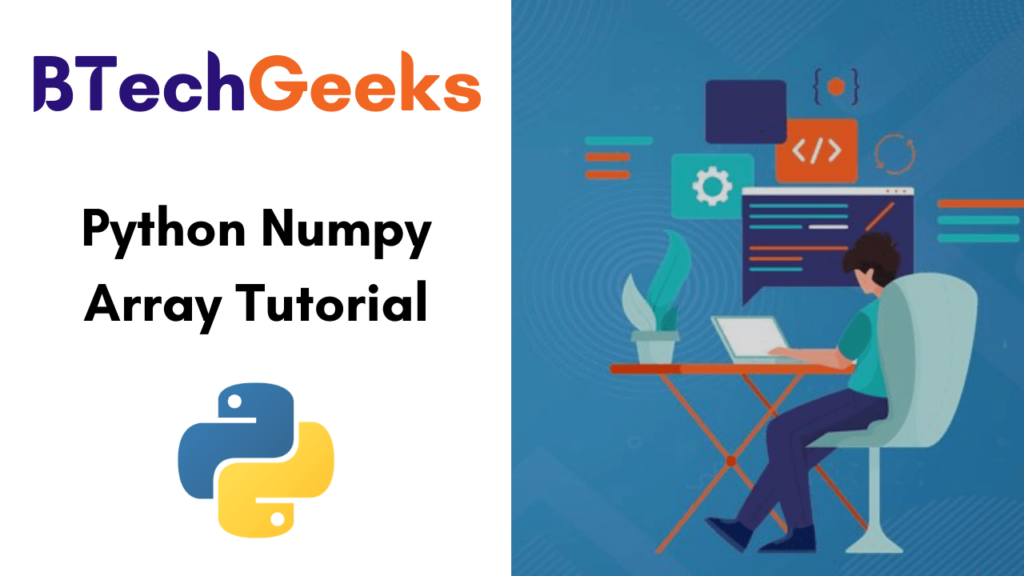# Python Numpy Array Tutorial for Beginners | Learn NumPy Library in Python – Complete Guide

In 2005, the NumPy package was created by Travis Oliphant with infusing the characteristics of the ancestor module Numeric into another module Numarray. Today, we are going to discuss everything regarding NumPy Array in Python Programming. BTech Geeks Python NumPy Array Tutorial Pdf aids beginners and professionals to learn the basics to advance core fundamentals of NumPy such as Creating, Searching, Selecting Elements, modifying, converting, and more.

## What is NumPy in Python?

NumPy is an open-source numeric python library that deals quite comfortably with multi-dimensional arrays and matrix multiplication. Also, the library of numpy performs mathematical and statistical operations in Python and aids in the programming of mathematical, scientific, engineering, and data science.

Moreover, easy integration with C/C++ and Fortran. You can also work in the creation of the N-dimensional array, linear algebra, random number, Fourier transform, etc. Look for more information from the below available NumPy related tutorials.

## What is Python NumPy Array?

It is the core library for scientific computing, and it stands for Numerical Python. One of the powerful N-dimensional array objects is in the form of rows and columns is called the Python NumPy Array. From the lists of nested python, we can start NumPy arrays and also access its elements.

In a Numpy array, the axis is the elements and separated by the same amount of bytes in the memory. To perform NumPy Array Operations, we need to install Numpy first. Also, it has functions to work in the domain of linear algebra, matrices, and Fourier transform.

### Why Use NumPy?

Unlike others, NumPy is fast, more memory efficient, convenient to work with matrix multiplication and reshaping. On the other hand, TensorFlow and Scikit study to utilize NumPy array for calculating the matrix multiplication in the back end.

Do Refer:

### Where is NumPy used?

NumPy Arrays in Python offer tools to integrate C, C++, etc. So, it is helpful in linear algebra, random number capability, and many more. Also, you can use this numpy array as an effective multi-dimensional container for common data.

### Benefits of Choosing NumPy Array in Python

However, you can observe many convincing arguments for learning and grasping this new standard. Here, we have mentioned the top four benefits of NumPy that can get into your code:

1. More speed: It applies C language written algorithms that perform in nanoseconds instead of seconds.
2. Fewer loops: You can reduce loops and avoid getting tangled up in iteration rules by NumPy.
3. Clearer code: Without loops, the program will seem like the equations you’re seeking to determine in NumPy.
4. Better quality: NumPy is quick, friendly, and bug-free as there are thousands of contributors working behind it.

### Which Language is NumPy Used in Programming?

NumPy is a library and is composed somewhat in Python, however, the majority of parts that need fast computation are written in C or C++ language.

### How to create a NumPy array?

We have many ways to create numpy arrays in python but now we are going to create an array using arrange() and shape() Numpy Array methods. Let’s, check the below example and learn how to create NumPy Arrays:

import numpy as np

A = np.arange(4)
print('A =', A)

B = np.arange(12).reshape(2, 6)
print('B =', B)

Output:

A = [0 1 2 3]
B = [[ 0 1 2 3 4 5]
[ 6 7 8 9 10 11]]

### Python NumPy Array Examples

Single-dimensional Numpy Array:

import numpy as np
a=np.array([41,62,53])
print(a)

Output: [41 62 53]

Multi-dimensional Array:

a=np.array([(1,2,3),(4,5,6)])
print(a)

Output: [[ 1 2 3]
[4 5 6]]

### NumPy Array Python Interview Questions for Freshers

The list of top 10 interview questions of python numpy array are given here for helping job seekers to prepare well and crack the interviews effectively.

1. What is the Numpy array?
2. Why should we use Numpy rather than Matlab, Idl, octave or Yorick?
3. How can you identify the datatype of a given NumPy array?
4. How can you Install NumPy on Windows?
5. What are the various features of NumPy?
6. List the steps to create a 1D array and 2D array
7. What is the difference between matrix and array?
8. How do you check for an empty (zero Element) array?
9. Create a two 2-D array. Plot it using matplotlib
10. What are the uses of NumPy?